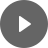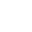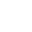12. Bivariate Data Analysis - StudyNinja
Select Page

HSC Standard Maths Resources

Browse: 1. Home  » 12. Bivariate Data Analysis

12. Bivariate Data Analysis

Check out the overview section for relevant importance of this section compared to other topics in this course. In 2020 exam, two questions appeared from this topic making up 6 marks (2020Q122020Q36). This section covers the following parts of the syllabus:

The infographic below shows all the past exam questions from 2010 to 2020 relevant to this topic sorted by difficulty level and further broken down into sub-topics. This will form the foundation of our study as we would like you to focus first on the easy questions and quickly develop skills to get those easy marks and then challenge yourself with the harder ones.This section explores the relationship between two variables for which we are given a few data points and don’t know about the relationship that exists between them.

The questions that are of interest when exploring this relationship are:

• How do the data points look on a scatterplot and if there is a visible relationship from eyeballing? (Discussed under ‘Scatterplot’)
• If it seems there is a relationship what is the strength of that relationship in quantitative terms? (Discussed under ‘Correlation’)
• If there exists a line that best defines this relationship, what is that line and how it can be used to predict? (Discussed under ‘Best Fit Line’).

Scatterplot:

Scatterplot is a representation of two variables on a graph in the form of dots.The following charts show different types of relationships:Sometimes you will find a point on scatterplot which stands out from the otherwise explainable relationship. These points are called outliers.

Example 1

The following table shows the study hours versus marks for 20 students. Draw it on scatterplot and comment on the relationship and determine and explain any outliers.Correlation Coefficient:

In the previous section, the relationship was determined by eyeballing and then categorized as strong, moderate or weak. Eyeballing may not work well sometimes when the two scatterplots look similar with little difference so there needs to be a standard way of quantifying this relationship which should make comparison easier.

This is called Pearson correlation coefficient (r).Example 2

Which one of the following charts is most likely to be associated with these correlation coefficients; i) 1 ii) -0.3 iii) 0.6 iv) -0.75?Best Fit Line:

Knowing that relationship exists between variables and knowing its strength leads to the next question as to if there is a way to define this relationship.

Best fit line is a line that, as the name suggests, fits these dots in the best possible way. By best possible way it means that the overall difference between the points and the line is minimized.

For example, it may look something like following where it can’t have all points on scatterplot on the line but overall the distance between line and each point is the least possible among all the ways line can be drawn.Once this line is determined, it can then be used to determine values that are not in the existing dataset and can be used as a predictor for the dependent variable.

Example 3

Best fit line for the following data has equation Weight = 1.3421 x Height – 157.82. Draw the scatterplot along with this line and determine what is the weight of person with height of 152cm as per the line? Would it be reasonable to use the equation to determine weight of person with height of 190cm?Get access to 20 Mock Exams with over 700 exam-style questions for HSC Standard Maths.

You might find the following videos helpful related to this section:6A Introduction to Bivariate Scatterplots (1 of 2)

by Mr Bodgers (click to view channel)

Introduces bivariate scatterplots. Completes an example, describing the correlation between a person's height and arm span.6A Introduction to Bivariate Scatterplots (2 of 2)

by Mr Bodgers (click to view channel)

Completes an example on bivariate scatterplots. The example shows the correlation between study time and exam marks.6B Bivariate Data Relationships (1 of 4)

by Mr Bodgers (click to view channel)

Explains how to describe the strength, form and direction of relationships when referring to scatterplots6B Bivariate Data Relationships (2 of 4)

by Mr Bodgers (click to view channel)

Completes an example where we describe the strength, form and direction of scatterplots.6B Bivariate Data Relationships (3 of 4)

by Mr Bodgers (click to view channel)

Explains the difference between dependent and independent variables when referring to scatterplots.6B Bivariate Data Relationships (4 of 4)

by Mr Bodgers (click to view channel)

Completes and example where we construct a scatterplot and describe the strength, form and direction of the scatterplot.6C Pearsons Correlation Coefficient (1 of 2)

by Mr Bodgers (click to view channel)

Defines Pearson's correlation coefficient and how it can be used to describe the strength and direction of a bivariate relationship.6C Pearsons Correlation Coefficient (2 of 2) Casio Calculator

by Mr Bodgers (click to view channel)

Completes an example where we find Pearson's correlation coefficient using a Casio calculator.6C Pearsons Correlation Coefficient (2 of 2) Sharp Calculator

by Mr Bodgers (click to view channel)

Completes an example where we find Pearson's correlation coefficient using a Sharp calculator.6D Line of Best Fit (1 of 2)

by Mr Bodgers (click to view channel)

Explains how to draw a line of best fit by eye and then use this to make predictions.6D Line of Best Fit (2 of 2) Casio Calculator

by Mr Bodgers (click to view channel)

Completes an example where we calculate the least squares line of best fit using a Casio calculator.6D Line of Best Fit (2 of 2) Sharp Calculator

by Mr Bodgers (click to view channel)

Completes an example where we calculate the least squares line of best fit using a Sharp calculator.6E Interpolation and Extrapolation (1 of 2)

by Mr Bodgers (click to view channel)

Expands on our knowledge of interpolation and extrapolation.6E Interpolation and Extrapolation (2 of 2)

by Mr Bodgers (click to view channel)

Completes an example that shows the inaccuracies of extrapolation.The following are the types of questions you can expect in exam:

Study notes of this section and other resources can be accessed here:

Browse: 1. Home  » 12. Bivariate Data Analysis